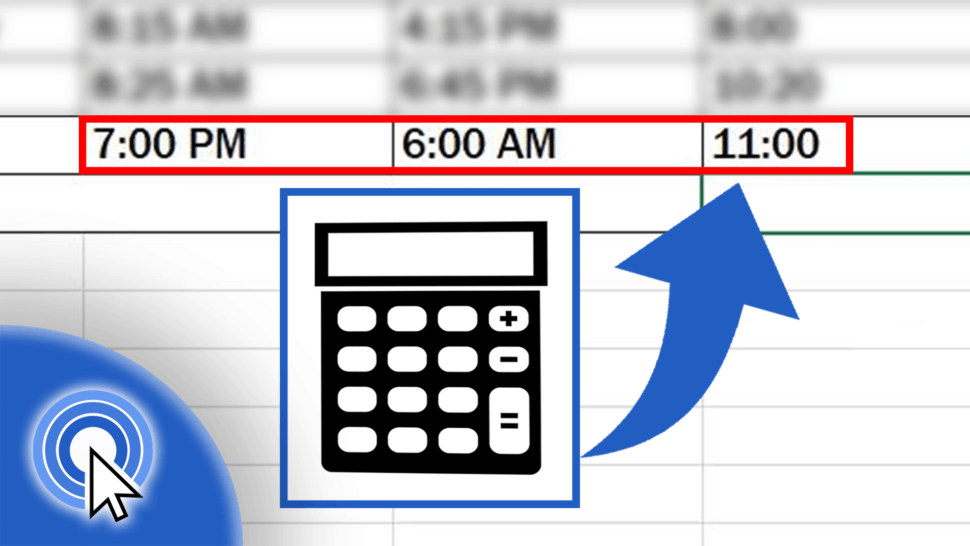# How to Calculate Hours Worked in Excel (Midnight Span)Together we’ll carry on with the tutorial on how to calculate hours worked after a night shift. There’s a specific formula to total hours worked past midnight in Excel, but after reading this tutorial, it’ll be a piece of cake!

Let’s get started!

See the video tutorial and transcription below:

In the last tutorial, we had a look at how to calculate hours worked if the ‘Start Work’ and ‘End Work’ times are within the same day. We also calculated working time for each day, from Monday to Friday. The problem is, that this usual way of calculating hours will not work for you if the working time spans midnight, which is the case of Friday.

On Friday, work started at seven o’clock in the evening and finished the next day, at six o’clock in the morning. Since the working time spanned midnight, which means the employee didn’t finish work on the same day, we need to use the function IF to calculate the hours correctly.

Let’s do this together!

## How to Use If Function in Excel

First, we need to remove the misfired attempt and, as usual, we type the ‘equal’ sign in the cell that is supposed to show the result. Now select the function IF by typing the first letter ‘I’ and double-click on IF once you see the options.

As you can see, for the function to work we need to enter the logical test first. Then we tell Excel what value to work with if the condition we entered is true, and right after that we need the value Excel should take into account if the result of the logical test is false.

In this tutorial, I’ll guide you step by step through how to work with the IF function in case you need to calculate time. More details on how the function works can be found in the video on how to use the IF function in Excel.

So, as for the beginning, we need to enter the logical test or condition based on which Excel will decide what value to use in calculation. After the ‘if’ word, we’ll start typing out the condition itself – the ‘Start Work’ time (the value in cell C7) is greater than the ‘End Work’ time (the value in cell D7), which is true for the working time that spans midnight.

Type in the comma and we’ll move on.

Now we need to indicate what value Excel should work with if the condition we entered is true. After that, we’ll also define the value for the situation in which the condition is false.

So, if the condition is true, we’ll tell Excel that the work was finished the next day. We’ll do so by clicking on the cell with the ‘End Work’ time data and we’ll add “+1”, which means that this time belongs to the next day.

Enter a comma again. Now we can type in the value Excel will use if the condition we entered at the beginning is false. If it’s false, we’ll let Excel know that the ‘End Work’ time is in the same day. This is done by clicking on cell D7. Excel will then treat the time data from cell D7 as it appears, as it was entered.

This specific condition is false for example for the Thursday entry, because the ‘End Work’ time is not greater than the ‘Start Work’ time. The formula we’re now entering will be valid for all cases – hours worked on the same day as well as working time that spans midnight. We can do a quick test to check that together at the end of the tutorial.

For now, we’ll carry on creating the formula.

Close the brackets.

Excel will now work as follows: if the value in C7 is greater than the value in D7, the value in D7 is from the next day. If this is not true, the value in D7 is from the same day and Excel will use this value as it is.

Now just subtract the ‘Start Work’ time by using the minus sign, click on cell C7 and press ‘Enter’. This is it!

You can see that now the calculated time (eleven hours) is accurate.

And because you should be able to use the formula for the cases of hours worked past midnight but also hours worked within the same day, here’s to check whether it works.

The formula in cell E7 can be copied through dragging the bottom-right corner of the cell up through all weekdays.

As you can see, the formula is in all the cells and the times calculated previously did not change. This means the formula works even in cases where the hours worked did not span midnight.

To see how we can easily add up hours worked throughout the whole week watch the next EasyClick Academy video tutorial!

If you’re interested, detailed steps on how to total hours along with the solution to common issues when calculating time are described in our video tutorial on how to sum time in Excel.

If you’ve found this tutorial helpful, like us and subscribe to receive more videos from EasyClick Academy. Look at more tutorials that help you use Excel quick and easy!

See you in the next tutorial!